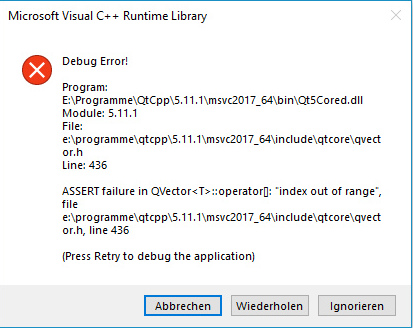# Problems with quickSorting a QVector

• Hello,

i've some Problems in my Project with quickSorting a QVector.

Its just a simple pointer QVector and i want to sort it .... strangely the sort Algorithm works in another projekt very good ...

and SOMEHOW sometime it works, sometimes NOT .... thats so strange o0

Error Pic:Error MSG:
ASSERT failure in QVector<T>::operator[]: "index out of range", file e:\programme\qtcpp\5.11.1\msvc2017_64\include\qtcore\qvector.h, line 436
16:31:09: The program has unexpectedly finished.
16:31:09: The process was ended forcefully.
16:31:09: [...] crashed.

You can find the whole project here:
https://github.com/Makozer/NpPartitionManager

The Code: (see vectorstash.cpp )

``````// Base Class
class VectorStash {
typedef QVector<Coin*> CoinList;
public:
[...]
void quickSortDesc();
private:
[...]
void quickSortDesc(quint16 leftpos, quint16 rightpos);
CoinList coins;
};

// GUI just uses this simple method
void VectorStash::quickSortDesc() {
if (coins.size() > 1) {
quickSortDesc(0, (coins.size() - 1));
}
}

// this method is called by the simple method
void VectorStash::quickSortDesc(quint16 leftpos, quint16 rightpos) {
quint16 i = leftpos, j = rightpos;
Coin* tmp;

int pivot = coins[(leftpos + rightpos) / 2]->getValue();

while (i <= j) {
while (coins[i]->getValue() > pivot) { i++; }
while (coins[j]->getValue() < pivot) { j--; }
if (i <= j) {
tmp = coins[i];
coins[i] = coins[j];
coins[j] = tmp;
i++;
j--;
}
};
/* recursion */
if (leftpos < j) { quickSortDesc(leftpos, j); }
if (i < rightpos) { quickSortDesc(i, rightpos); }
} // end quickSortDesc
``````

I cant see or find why im getting a "index out of range" error :(

the next problem is, as a new it student im used to programm on the console and im able to print me all useful steps so i can see where it crashes, here the real big problem is that i just get this error msg and program shuts down, no way to see better information on this bug ...

debugger wont works, too :/

does someone sees the problem? or has a useful tip how i can do bette rresearch on this bug?

thx =)

greetings

• Hi
In GUI programs you can use
qDebug() << "Text=" << variable;
which shows up inside qtCreator.

• Just delete your sort implementation. Why would anybody waste time implementing a custom sort?
if `coins` is of type `QVector<Coin*>` just use `std::sort(coins.begin(),coins.end(),[](Coin* a, Coin* b)->bool{return return a->getValue() < b->getValue() ;});`

• Hi
In GUI programs you can use
qDebug() << "Text=" << variable;
which shows up inside qtCreator.

thanks!!! i will try it =D

Just delete your sort implementation. Why would anybody waste time implementing a custom sort?
if `coins` is of type `QVector<Coin*>` just use `std::sort(coins.begin(),coins.end(),[](Coin* a, Coin* b)->bool{return return a->getValue() < b->getValue() ;});`

well, because we had to learn the algorithm in one module and i wanted to fully understand it so i wrote it :D
and i need it ascending and descending, depending how i choose, is that possible with std::sort? i guess so? :D
oh wait maybe i can do it in that case ... ill have a short look